mso-para-margin:0cm; mso-para-margin-bottom:.0001pt; mso-pagination:widow-orphan; font-size:12.0pt; font-family:"Times New Roman";}

### Abstract

In the paper [a] the real options theory and its practical use as a tool to estimate the value of small companies, research projects and patent portfolios are described. In particular small or very small companies are considered. Here the term “small company" means a company that produces only one product or a few products and that can be satisfactorily modeled with a few variables. The economic value of these companies is of great interest in the Italian economic context since this context is characterized by a multitude of small companies. Furthermore due to the globalization process, the aggregation of these small companies is becoming necessary to compete in the international markets. Moreover the international context forces the Italian companies to re-qualify their product portfolios making relevant the problem of the quantitative evaluation of research projects, patent portfolios and so on. The real options methodology may be a practical tool to get these quantitative evaluations. This website contains some illustrative material and two interactive applets that are supposed to help the understanding of [a].

[a] P. Corna, L. Fatone, M.C. Recchioni, F. Zirilli, Le opzioni reali come strumento di valutazione di piccole imprese e di progetti innovativi, Congiuntura 2 (2005) 77-97.

### References


Amram M., Kulatilaka N. (2000), Strategy and shareholder value creation: the real options frontier, Journal of Applied Corporate Finance, 13, 8-21.

Amram M., Kulatilaka N. (1999), Uncertainty: The new rules for strategy, Journal of Business Strategy, 20, 25-29.

Black F., Scholes M. (1973), The pricing of options and corporate liabilities, Journal of Political Economy, 81, 637-659.

Brennan M., Trigeorgis L. (2000), Project flexibility, agency and competition, Oxford, Oxford University Press.

Copeland T. (2001), Real options a practitioner’s guide, New York, Texere LLC.

Myers S. (1977), Determinants of corporate borrowing, Journal of Financial Economics, 5(2), 147-175.

Schwartz E.S. (2004), Patents and R&D as real options, NBER (National Bureau of Economic Research) working paper n. W10114, http://papers.nber.org/papers/w10114.pdf

Trigeorgis L. (1993), Real options and interactions with financial flexibility, Financial Management, 22, 202-223.

Trigeorgis L. (1990), A real options application in natural resource investments, Advances in Futures and Options Research, 4, 153-164.

### Real options

In this website we provide the user with a tool to price financial options and real options in the circumstances when the problem of determining the real option price can be reduced to the problem of determining the price of an European call or put financial option. In order to make easy the use of this website we give a brief decription of the formula to price an European call financial option in the so called Black and Scholes model. This formula is known as Black-Scholes formula . The name financial option is due to the fact that it is assumed that the asset underlying the option contract is an asset traded on the financial market (equity,...). In the following we write option to mean financial option.

An European call option on a single asset is a contract between two sides, i.e.: the owner and the writer. The writer gives to the owner the right but not the obligation to purchase at a given time in the future, the expiry date or maturity time, a prescribed quantity of a given asset or underlying at a given price known as exercise price or strike price. Since the option confers a right to the owner, the owner must pay to the writer an amount of money for the right acquired at the time when the contract is drawn up. This amount of money is the option price. Let us denote with S, K, T the price of the underlying, the strike price, the expiry date respectively and finally let us denote with V(S,t), S >0, t < T the option price as a function of the underlying price S and of the time t.

The Black-Scholes formula for pricing the European call option is based on the following assumptions on the behaviour of the market of the underlying:

a)               the trading of the underlying takes place continuously in time;

b)               it is possible buying and selling any fraction of the underlying;

c)               there are no transaction costs in buying or selling the underlying;

d)               the risk free interest rate is a known constant value;

e)               there are no arbitrage possibilities.

Finally the Black Scholes formula is based on the assumption that the price of the underlying satisfies the following stochastic differential equation:

(1)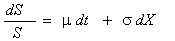,

where s, m are two constants, s > 0,  t is the time variable,   X(t) is  a standard brownian motion and dX is its stochastic differential. The value of an European call  option is known at the expiry time and is given by the following formula:

(2)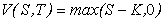,     S > 0 ,

where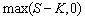denotes the maximum value between S-K and 0. Moreover we assume K >0.

The function appearing in formula (2) is known as payoff function of an European call option. Black and Scholes in  proved that the value V(S,t), S >0, t < T, of an European option satisfies the following partial differential equation:

(3)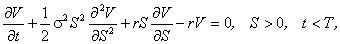where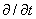denotes the first order partial derivative with respect to the time variable and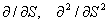denote the first and second order derivatives with respect to S respectively and r denotes the risk free interest rate. Equation (3) equipped with the final condition (2) is the Black Scholes mathematical model for pricing European call options. This model has an explicit solution known as Black-Scholes formula , that is:

(4)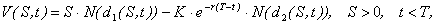where

(5)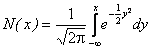,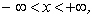and

(6)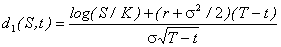,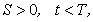(7)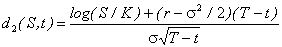,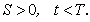A formula similar to formula (4) can be derived for European put options. In fact in this case it is necessary to susbstitute (2) with:

(8)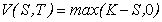,    S > 0.

and to solve the model given by (3), (8).

In this website we consider the following real options: postponement option, abandonment option, expansion option and contraction option. These options are referred generically to the management of projects or investments, the project or investment considered may be a small company or a research project or a patent portfolio.

A postponement option gives to its owner the possibility to postpone the beginning of an investment to a fixed time in the future. Thank to this postponement period the owner can get information useful to the management of the investment. This option can be interpreted as an European call option on the project value. This option gives the right but not the obligation to make an investment paying an exercise price. The underlying of this real option is the present value of the gross cash-flow deriving from the project when the project is active and the exercise price is the cash necessary to start the project.

An abandonment option allows its owner to abandon at a fixed time T in the future an investment when the cash-flow generated by the investment is smaller than the expected cash-flow. When the owner abandons the investment he receives a value that mitigates the loss of the cash-flow deriving from the abandonment. At time T, the owner has two alternatives: not to exercise the option (hence to continue the management of the investment since it is producing the expected cash-flow) or to exercise the option getting the abandonment value to mitigate the loss due to the abandonment of the investment. This option can be used to protect the capital employed in the investment against the market risk. This option is similar to an European put option.

An expansion option allows its owner to increase the amount of the investment at a future date if market conditions become more favourable than the initial market conditions. The owner of the option can either mantain the initial amount invested in the project or expand the project investing more money at the future fixed date. This kind of option can be interpreted as an European call option where the exercise price is the extra amount necessary to expand the project and the payoff function is given by equation (2).

A contraction option is exactly the mirror case of the expansion option. In fact the contraction option allows the owner to reduce the volume of the production or the dimension of a project or of an investment when the market conditions turn against the interest of the owner. The contraction can be realized, for example, selling a portion of the project at a fixed value K. Hence a contraction option is substantially an European put option with K as exercise price and the present value of the gross cash-flow that can be generated by the project after the contraction as underlying.

Summing up the real options presented in this website can be modeled as European call or put options according to Table 1.

TABLE 1
 Real option Payoff Postponement option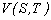=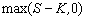( call option) where T = end of postponement period, K = initial investment, S = present value of the gross cash-flow       generated by the project. Abandonment option=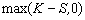(put option) where T =abandonment time, i.e. time at which,       the renouncecan take place, K =recovery value, S =present value of the gross cash-flow           generated by the project. Expansion option=( call option) where T = expansion time, i.e time at which one can       exercises the option, K = additional amount of the investment, S = actual value of the additional gross          cash-flow generated by the project. Contraction option=(put option) where T =contraction time, i.e. the time at which one       can exercise the contraction option, K = renounced amount of the investment, S = actual value of the gross cash-flow         that can be generated by the project          after the contraction.

The use of the Black Scholes model in the context of real options pricing is based on the assumption that the hypotheses of the Black Scholes model hold in the real option context.

The interactive material contained in Applet 1 can be used to price financial and real options assigning the following input parameters:

S=price of the underlying (0<S<10000),

K=exercise price (0<K<10000),

s=underlying volatility (0<s<1),

r=risk free interest rate (0<r<1),

t=T-t=time to maturity (0<t<10).

The option prices are obtained using the Black Scholes model.

The interactive material contained in Applet 2 allows the user to analyze the behaviour of the price of European financial or real options as function of the price of the underlying for several values of the time to maturity. The option prices are obtained using the Black Scholes model. The user must provide the following input parameters:

Smin=smallest value of the underlying price interval considered in the simulation (Smin>0),

Smax=largest value of the underlying price interval considered in the simulation (Smax<100000, Smin < Smax),

K=exercise price (0<K<10000),

s=underlying volatility (0<s<1),

r=risk free interest rate (0<r<1),

tmax=T-tmin=maximum time to maturity considered (0<tmax<10),

N= number of time steps where the user desires to look at the option price graph as a function of the underlying price S (0< N <51), that is Applet 2 shows the graphs V(S,T- itmax/N), Smin<S< Smax, i=1,2,...,N.

In the Applets 1 and 2 default values are assigned to the parameters that must be assigned by the user. This is done to make possible the use of the Applets to everybody.

We conclude specifying the units of measure of the quantities used in the two Applets. In details, S has the dimension of a monetary unit, K has the dimension of a monetary unit, s2 has the dimension of the inverse of a time, r has the dimension of the inverse of a time, and finally t=T-t has the dimension of a time. Indeed the time is measured in years or fractions of year, that is t=1 denotes one year.

Entry n. 1076